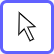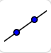# Different Types of Slopes

## Click on the line tool and then click two places on the grid to draw a line.Move toolLine tool

## Use the line tool to draw a line that has a positive slope that is VERY close to a slope of zero.

If you give this slope an approximate numerical value, what would it be?

Check all that apply

## Use the line tool to draw a line that has a negative slope that is very close to a slope of zero.

If you give this slope an approximate numerical value, what would it be?

Check all that apply